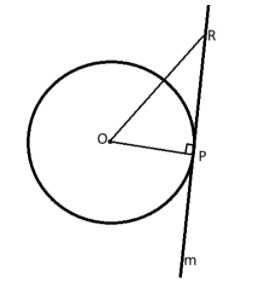How many tangents can be drawn to a circle from a point on the same circle? Justify your answer.Verified
83.8k+ views
Hint: Only one tangent can be drawn to a circle from a point on the same circle, we will prove this by constructing a line perpendicular to the radius and will prove that all other points of the line lie exterior of the circle and hence touches the circle at a single point.

Complete step by step solution: Suppose a circle with centre ‘O’ and a point ‘P’ on the circle and make a line perpendicular to OP, let say a line(m).
Now take any point ‘R’ on the line m,We can say that${\text{OP < OR}}$ since the perpendicular distance is the shortest, we can say that ‘R’ is an exterior point and lie outside the circle and this will be true for all points lying on line(m) except ‘P’.
Since line(m) touches the circle at a single point, we can say that tangent at any point of a circle is unique.

Note: We can also prove that be the statement that tangent at any point shows the slope of that point as regarding the positive x-axis. Since the slope of a line can never have different values so tangent to a point of a circle is unique.